# Marks at school

There are 30 students in the class. Five students in the class had a mark three triple at the end of the math certificate, and the other students had a mark of one or two. The average mark in all students' mathematics in the class at the end of the year was 1.9. Find out how many students in the class had a mark one on the year-end math certificate.

x =  8

### Step-by-step explanation:

1•x + 5•3+ (30-5-x)•2= 1.9•30

x = 8

Our simple equation calculator calculates it.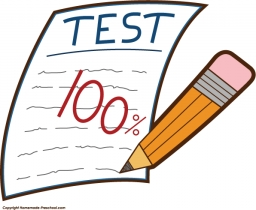Did you find an error or inaccuracy? Feel free to write us. Thank you!Tips to related online calculators
Looking for help with calculating arithmetic mean?
Looking for a statistical calculator?
Do you have a linear equation or system of equations and looking for its solution? Or do you have a quadratic equation?

#### You need to know the following knowledge to solve this word math problem:

We encourage you to watch this tutorial video on this math problem:

## Related math problems and questions:

• Certificate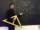There is 31 students in a class. From mathematics was'nt worse mark than 2. Average mark in mathematics was 2. How many students have mark 1 and how many mark 2?
• Exam average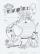The average of marks that have on the certificates students of 8A class in mathematics is exactly 2.45. If we did not add 1 and 3 of students Michael and Alena, who arrived a month ago, it would average exactly 2.5. Determine how many students have class
• Classs meanClass size is 30 students, math was not worse than sign 2. Determine the number of pupils who were sign 1 if the class had a 1.4 sign average.
• In the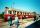In the 6th class, the average half-year mark in physics was 1.7. At the end of the year, Maťo improved his mark from 2 to 1, Ivanka from 3 to 2, and Elenka from 4 to 2. Pavlík worsened his mark from 3 to 4. The average mark of the whole class thus improve
• Class 9AOn the final certificate have one quarter of the class 9A mark "C" of mathematics, seventh mark "C" from the Czech language and two students failed in chemistry. How many students attend class 9A?
• Holidays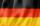Of the 35 students of the class were 7 on holiday in Germany and just as much in Italy. 5 students visited Austria. In none of these countries was 21 students, all three visited by one student. In Italy and Austria were 2 students and in Austria and Germa
• Prospering studentsThere are girls and 30 boys in the class. Boys prospering 28, girls all. How many girls are in the class and how many boys are there, if all the prospering students are 95%?
• Average score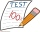In a math exam, two students scored 95, six students scored 90, 3 students scored 80, and one students scored 50. What was the average score for the class?
• Average height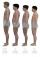In a class are 34 students. The average height of the students is 165 cm. What will be the average height of students in the classroom when two pupils, tall 176 cm and 170 cm, moved from this school/class? The average height in meters of all the students
• 9.A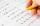9.A to attend more than 20 students but fewer than 40 students. A third of the pupils wrote a math test to mark 1, the sixth to mark 2, the ninth to mark 3. No one gets mark 4. How many students of class 9.A wrote a test to mark 5?
• Three subjects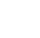In a class of 40 students, 18 passed mathematics, 19 passed accounts, 16 passed economics, 5 mathematics and accounts only, 6 mathematics only, 9 accounts only, 2 accounts and economics only, if each student offered at least one of the subjects. a) how ma
• Target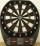Peter, Martin and Jirka were fire in a special target, which had only three fields with values of 12, 18 and 30 points. All boys were firing with the same number of arrows and all the arrows hit the target, and the results of every two boys differed in on
• In the classroomThere are 30 boys and a few girls in the class. In the six months, 28 boys and all girls benefited, which was 95% of all pupils. How many pupils are there in the classroom?
• Ski class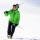Class attend 30 boys and some number of girls. Ski training was attended by 28 boys and all the girls, which was 95% of all students. How many girls attend this class? How many percent is that?
• Math testStudents wrote a math test. The average number of points obtained by them was 64. Another student wrote this test for 80 points. If the teacher added his result to the original ones, the total average of all students would be 65. How many students origina
• Average heightThere are twice as many girls in the class as there are boys. The average height of girls is 177 cm, boys 186 cm. What is the average height of students in this class?
• Average weightMom bought 6 apples with an average weight of 130 g - If she took 1 apple out of the bag, the average weight of the remaining apples changed to 140 g. What was the weight of the removed apple?